rfplot

Plot cumulative RF budget result versus cascade input frequency

Description

example

rfplot(rfobj,str) plots the RF budget result specified by STR versus a range of input frequencies. The input frequencies are applied to the cascade of elements in the RF budget object, rfobj.

Cumulative (that is, terminated subcascade) results are automatically computed to show the variation of the RF budget result through the entire design.

rfplot(rfobj) plots the magnitude response of S-Parameters, S21 for the cascaded budget object, rfobj.

rfplot(rfobj,m,n) plots the magnitude response of S-Parameters, Smn (S11, S12, S21 , or S22) for the cascaded budget object, rfobj.

Examples

collapse all

Create an RF system.

Create an RF bandpass filter using the Touchstone® file RFBudget_RF.

f1 = nport('RFBudget_RF.s2p','RFBandpassFilter');

Create an amplifier with a gain of 11.53 dB, a noise figure (NF) of 1.53 dB, and an output third-order intercept (OIP3) of 35 dBm.

a1 = amplifier('Name','RFAmplifier','Gain',11.53,'NF',1.53,'OIP3',35);

Create a demodulator with a gain of -6 dB, a NF of 4 dB, and an OIP3 of 50 dBm.

d = modulator('Name','Demodulator','Gain',-6,'NF',4,'OIP3',50, ...
'LO',2.03e9,'ConverterType','Down');

Create an IF bandpass filter using the Touchstone file RFBudget_IF.

f2 = nport('RFBudget_IF.s2p','IFBandpassFilter');

Create an amplifier with a gain of 30 dB, a NF of 8 dB, and an OIP3 of 37 dBm.

a2 = amplifier('Name','IFAmplifier','Gain',30,'NF',8,'OIP3',37);

Calculate the RF budget of the system using an input frequency of 2.1 GHz, an input power of -30 dBm, and a bandwidth of 45 MHz.

b = rfbudget([f1 a1 d f2 a2],2.1e9,-30,45e6)
b =
rfbudget with properties:

Elements: [1x5 rf.internal.rfbudget.Element]
InputFrequency: 2.1 GHz
AvailableInputPower: -30 dBm
SignalBandwidth:  45 MHz
Solver: Friis
AutoUpdate: true

Analysis Results
OutputFrequency: (GHz) [   2.1    2.1   0.07    0.07   0.07]
OutputPower: (dBm) [-31.53    -20    -26  -27.15  2.847]
TransducerGain: (dB)  [-1.534  9.996  3.996   2.847  32.85]
NF: (dB)  [ 1.533  3.064  3.377   3.611  7.036]
IIP2: (dBm) []
OIP2: (dBm) []
IIP3: (dBm) [   Inf     25  24.97   24.97  4.116]
OIP3: (dBm) [   Inf     35  28.97   27.82  36.96]
SNR: (dB)  [ 65.91  64.38  64.07   63.83  60.41]

Plot the available output power.

rfplot(b,'Pout')
view(90,0)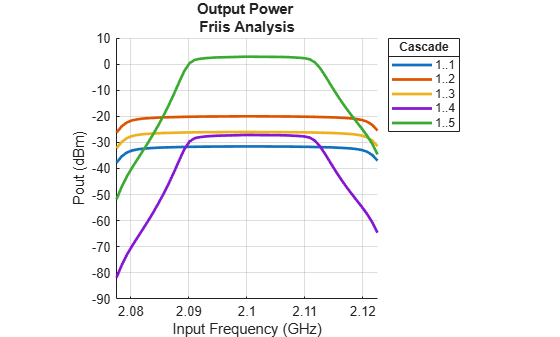Plot the transducer gain.

rfplot(b,'GainT')
view(90,0)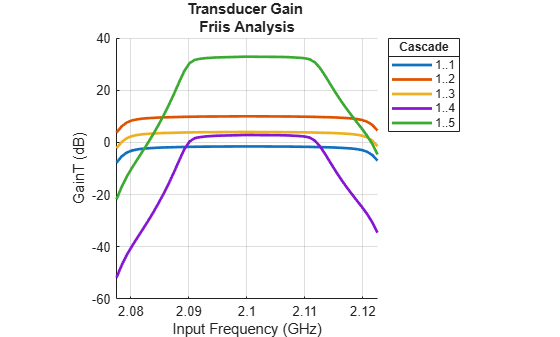Plot S-parameters of an RF system on a Smith Chart and a Polar plot.

s = smithplot(b,1,1,'GridType','ZY');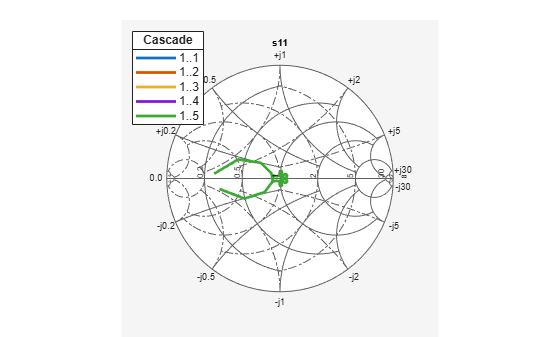p = polar(b,2,1);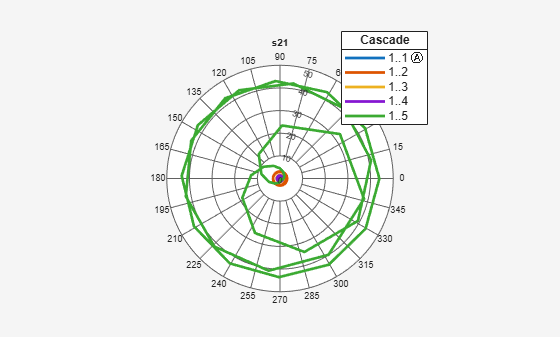Create an amplifier with a gain of 4 dB.

a = amplifier('Gain',4);

Create a modulator with an OIP3 of 13 dBm.

m = modulator('OIP3',13);

Create an nport using passive.s2p.

n = nport('passive.s2p');

Create an rf element with a gain of 10 dB.

r = rfelement('Gain',10);

Calculate the rf budget of a series of rf elements at an input frequency of 2.1 GHz, an available input power of -30 dB, and a bandwidth of 10 MHz.

b = rfbudget([a m r n],2.1e9,-30,10e6);

Show the analysis in the RF Budget Analyzer app.

rfplot(b)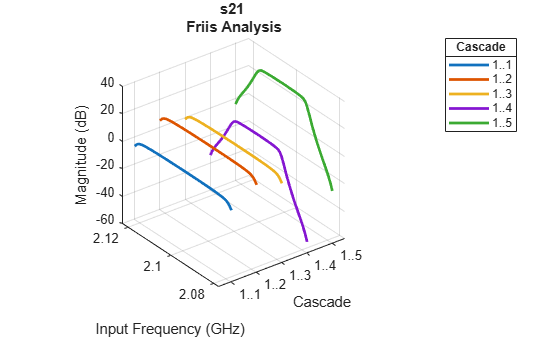Group Delay

To plot the group delay, first plot the S11 data for the RF System.

rfplot(b,1,1)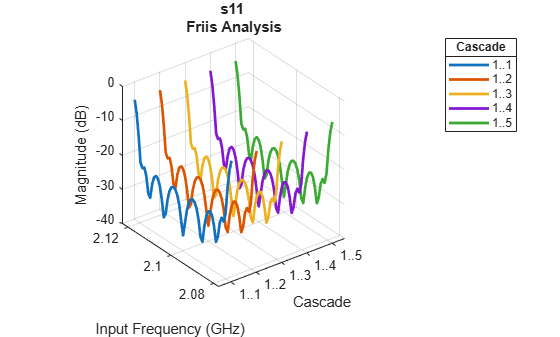Use the Group Delay option on the plot graph to plot the group delay of the RF system.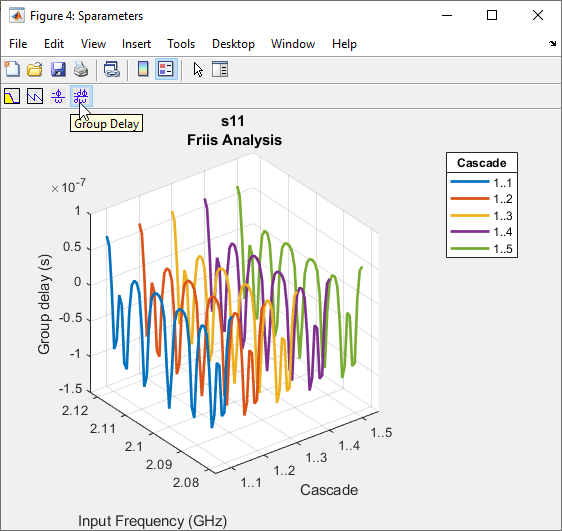Phase Delay

Use the Phase Delay option on the plot graph to plot the phase delay of the RF System.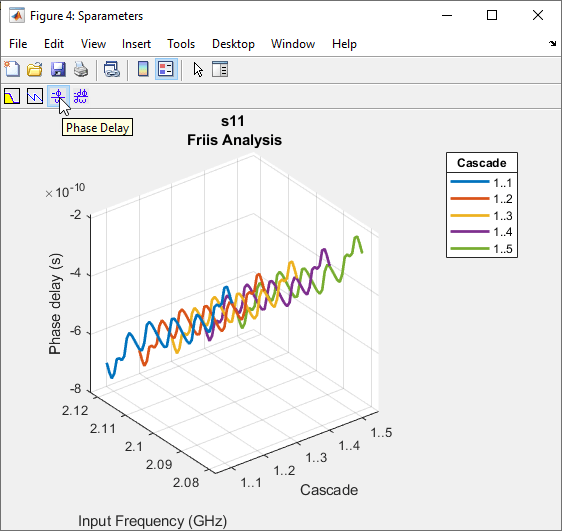Input Arguments

collapse all

Cumulative RF budget results, specified as an object.

Example: rfplot(rfobj,'Pout') where rfobj is created using rfbudget object.

STR values, specified as one of the following:

• 'Pout' - Available output power (dBm)

• 'GainT' - Transducer gain (dB)

• 'NF' - Noise Figure (dB)

• 'OIP3' - Output Third-Order Intercept (dBm)

• 'IIP3' - Input Third-Order Intercept (dBm)

• 'SNR' - Signal-to-Noise Ratio (dB)

• 'Sparameters' - S - Parameters S21 magnitude response (dB)

Example: rfplot(rfobj,'Pout') where 'Pout' is the available output power of an RF system obtained from the RF budget analysis.